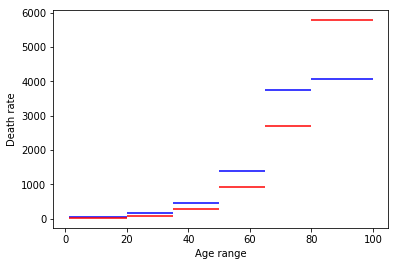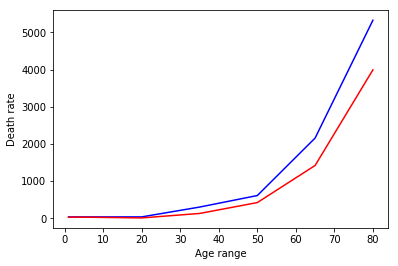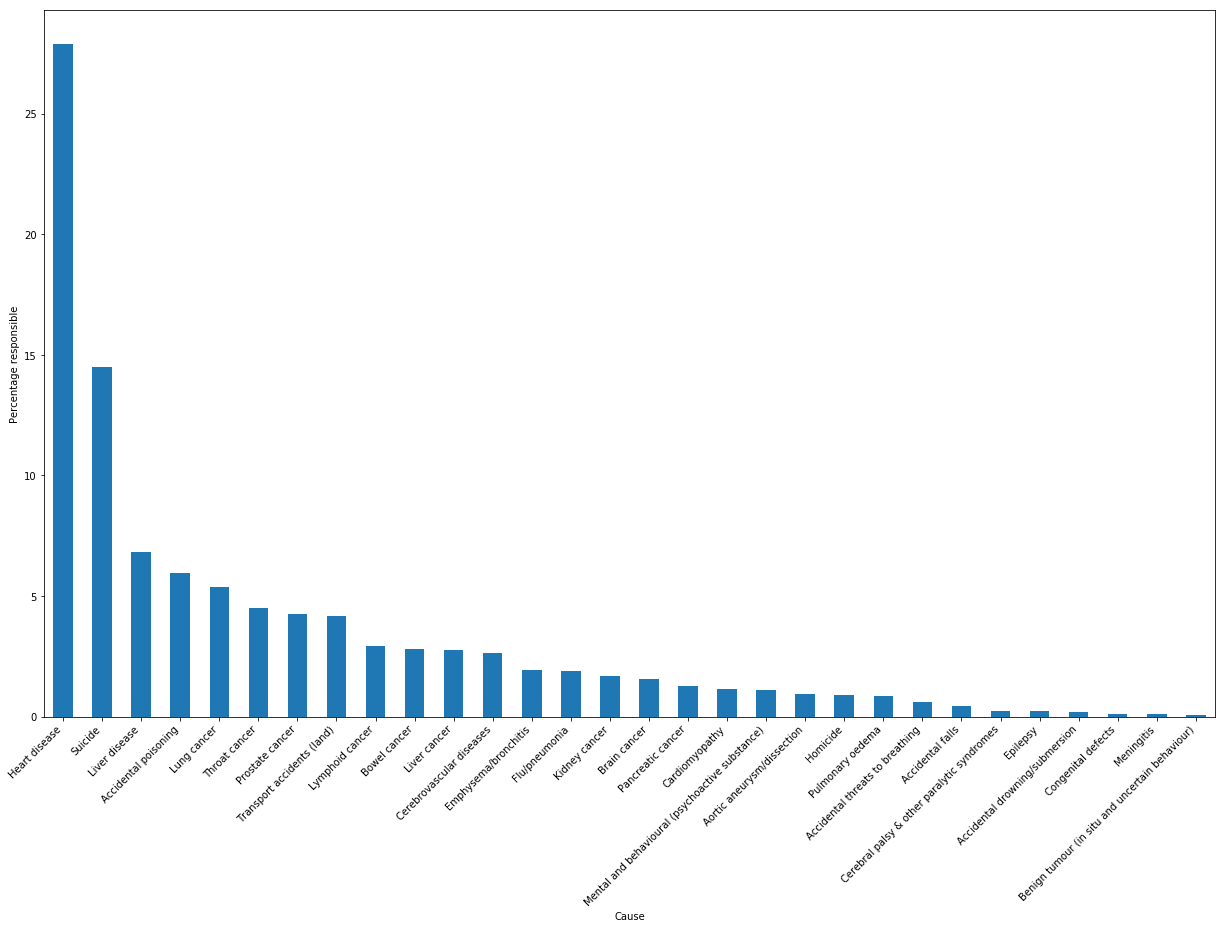# Causes of gender differences in life expectancy¶

The existence of a significant gap between men's and women's life expectancy in developed countries is well known, but there is little general quantitative research on its causes. In this analysis we take British mortality statistics from 2013, adjust male death rates from each cause in each age group to match respective female ones, and calculate the change in life expectancy this gives.

### Analysis¶

First, import the relevant Python modules and load the data.

In :
import copy
import numpy as np
import pandas as pd
import matplotlib
import matplotlib.pyplot as plt
import scipy
import scipy.optimize
%matplotlib inline

In :
df = pd.read_csv('https://visual.ons.gov.uk/wp-content/uploads/2015/02/Leading-Causes-of-Deaths-20131.csv', encoding='cp1252', header=None,
names=['ICD10 code', 'Displayed cause label', 'Cause of death', 'Number'])

males = []
females = []
ages = [(1,4), (5,19), (20,34), (35,49), (50,64), (65,79)]

males.append('Males All Ages')
females.append('Females All Ages')
for pair in ages:
males.append('Males {0}-{0} yrs'.format(pair))
females.append('Females {0}-{0} yrs'.format(pair))
males.append('Males 80+ yrs')
females.append('Females 80+ yrs')

table_names = males + females

df = df.dropna(how='all')
groups = df['ICD10 code'].isin(table_names).cumsum()
tables = {g.iloc[0,0]: g.iloc[2:] for k, g in df.groupby(groups)}

for k in tables:
tables[k]['Number'] = pd.to_numeric(tables[k]['Number'])

males.remove('Males All Ages')
females.remove('Females All Ages')
ages.append((80, 99))    # placeholder
ages = [(a, b+1) for (a, b) in ages]        # so graphs are continuous
tables['Males 5-19 yrs']

Out:
ICD10 code Displayed cause label Cause of death Number
39 V01-V89 Transport accidents (land) Land transport accidents 128
40 X60-X84,Y10-Y34 Suicide Suicide and injury/poisoning of undetermined i... 112
41 X85-Y09,Y33.9 Homicide Homicide and probable homicide 47
42 C81-C96 Lymphoid cancer Malignant neoplasms of lymphoid, haematopoieti... 42
43 Q00-Q99 Congenital defects Congenital malformations, deformations and chr... 38
44 G80-G83 Cerebral palsy & other paralytic syndromes Cerebral palsy and other paralytic syndromes 33
45 C71 Brain cancer Malignant neoplasm of brain 32
46 X40-X49 Accidental poisoning Accidental poisoning 29
47 W75-W84 Accidental threats to breathing Accidental threats to breathing 20
48 J40-J47 Emphysema/bronchitis Chronic lower respiratory diseases 18
49 W65-W74 Accidental drowning/submersion Accidental drowning and submersion 17
50 G40,G41 Epilepsy Epilepsy and status epilepticus 17
51 C40-C41 Bone cancer Malignant neoplasms of bone and articular cart... 17
52 A39,A87,G00-G03 Meningitis Meningitis 11
53 J09-J18 Flu/pneumonia Influenza and pneumonia 10

To calculate life expectancy from data given in age groups, we need to find the expected age of death for a person in each group. The obvious way to do this would be to average the endpoints of each range, but that would be inaccurate since older people within an age range are more likely to die. Instead, we assume the death rate is a piecewise linear function, find that function, and use it to calculate the expected ages of death for each range. Graphically, the simple method uses this as the death rate function:

In :
def get_rates_and_totals(gender, tables):
totals = [sum(tables[k]['Number']) for k in gender]
rates = [total / (b - a) for total, (a, b) in zip(totals, ages)]

m_totals, m_rates = get_rates_and_totals(males, tables)
f_totals, f_rates = get_rates_and_totals(females, tables)
start_ages, end_ages = zip(*ages)

plt.hlines(m_rates, start_ages, end_ages, color='b')
plt.hlines(f_rates, start_ages, end_ages, color='r')

plt.xlabel('Age range')
plt.ylabel('Death rate')
plt.show()When we actually want this:

In :
def calculate_edge_rates(gender_rates):
"""
Calculate all but the last edge rate.
"""
edge_rates = []
current = gender_rates    # assume that death rate in first category is flat (can't assume it starts at 0 and increases because range starts at age 1)
edge_rates.append(current)
edge_rates.append(current)    # duplication intentional
for i, _ in list(enumerate(gender_rates))[1:-1]:
new = 2*gender_rates[i] - edge_rates[-1]        # we want a trapezium with area totals
edge_rates.append(new)
return edge_rates

m_edge_rates, f_edge_rates = calculate_edge_rates(m_rates), calculate_edge_rates(f_rates)
m_xs, f_xs = start_ages[:], start_ages[:]

for xs, edge_rates, color in zip([m_xs, f_xs], [m_edge_rates, f_edge_rates], ['b', 'r']):
plt.plot(xs, edge_rates, color=color)

plt.xlabel('Age range')
plt.ylabel('Death rate')
plt.show()The final group is unbounded, so its distribution can't be calculated with the data we have (and probably couldn't be simply modelled using the same method for both genders anyway). So it should be treated separately, by just finding the mean that gives actual life expectancies (79.2 for men and 82.9 for women) and using that even after male death rates are adjusted. The mean of the other age groups can be found by scaling them to be probability density functions and integrating to get the expected value. Then the overall life expectancy is calculated by taking the weighted average of age group means.

In :
def age_range_mean(start_age, end_age, start_rate, end_rate, total_deaths):
# scaling to get p.d.f.
x = start_rate / total_deaths
y = end_rate / total_deaths

# rename for brevity
a, b = start_age, end_age

# use formula found by integrating equation of line
return (1/3)*(y - x)*(b**2 + b*a + a**2) + (1/2)*(x*b - y*a)*(b + a)

def life_expectancy(gender_xs, gender_edge_rates, gender_totals, last_segment_mean):
assert len(gender_xs) == len(gender_edge_rates) == len(gender_totals)
range_means = [age_range_mean(gender_xs[i], gender_xs[i+1],
gender_edge_rates[i], gender_edge_rates[i+1], gender_totals[i])
for i in range(len(gender_xs) - 1)]
# treat last segment specially
range_means.append(last_segment_mean)
assert len(range_means) == len(gender_totals)

return sum(np.array(range_means)*np.array(gender_totals)) / sum(gender_totals)

M_TARGET = 79.2
F_TARGET = 82.9

m_last_mean = scipy.optimize.fmin(lambda x: (life_expectancy(m_xs, m_edge_rates, m_totals, x) - M_TARGET)**2, 90, disp=0)
f_last_mean = scipy.optimize.fmin(lambda x: (life_expectancy(f_xs, f_edge_rates, f_totals, x) - F_TARGET)**2, 90, disp=0)
print('Male 80+ mean: {:.2f}, female 80+ mean: {:.2f}'.format(m_last_mean, f_last_mean))

Male 80+ mean: 93.48, female 80+ mean: 91.20


Check that we have calculated the 80+ mean correctly:

In :
m_unchanged = life_expectancy(m_xs, m_edge_rates, m_totals, m_last_mean)
f_unchanged = life_expectancy(f_xs, f_edge_rates, f_totals, f_last_mean)
print('Male life expectancy: {:.2f}, female life expectancy: {:.2f}'.format(m_unchanged, f_unchanged))

Male life expectancy: 79.20, female life expectancy: 82.90


These values are correct. Now actual life expectancies have been calculated, we adjust male death rates and see the effect on life expectancy.

In :
m_all = sum(tables['Males All Ages']['Number'])
f_all = sum(tables['Females All Ages']['Number'])

def change_cause(cause_label, age_range_index, working_tables):
"""
Return a new set of tables, where the male deaths due to cause_label
for the age range numbered age_range_index have been adjusted to match the matching female number.
"""
f_index = females[age_range_index]
f_living = f_all - sum(sum(tables[c]['Number']) for c in females[:age_range_index])
f_killed = sum(np.where(tables[f_index]['Displayed cause label'] == cause_label, tables[f_index]['Number'], 0))
f_proportion = f_killed / f_living
m_living = m_all - sum(sum(working_tables[c]['Number']) for c in males[:age_range_index])
new_m_killed = f_proportion * m_living
m_index = males[age_range_index]
new = working_tables[m_index].copy()
new['Number'] = np.where(new['Displayed cause label'] == cause_label, new_m_killed, new['Number'])
new_tables = copy.deepcopy(working_tables)
new_tables[m_index] = new
return new_tables

def match_rates(*cause_labels, stopping_offset=0):
"""
Change male death rates to match female ones for a given cause.
"""
new_tables = copy.deepcopy(tables)
for index in range(len(males) - stopping_offset):
for cause_label in cause_labels:
new_tables = change_cause(cause_label, index, new_tables)

return new_tables

def expectancy(tables, gender):
totals, rates = get_rates_and_totals(gender, tables)
edge_rates = calculate_edge_rates(rates)

if gender == males:
xs = m_xs
last_mean = m_last_mean
elif gender == females:
xs = f_xs
last_mean = f_last_mean

return life_expectancy(xs, edge_rates, totals, last_mean)


Given these functions, we can now adjust male death rates to match female ones and look at the effect of that on life expectancy. To get accurate results, we will not change the rates in the 80+ age range.

This is because our method of adjusting simply removes people whose deaths are eliminated from consideration, rather than adding them back in somewhere else. Most of the time, this produces a fairly accurate result, as it effectively involves assuming someone who died at e.g. 30 could have been expected to live to the general life expectancy, which is generally reasonable. However, for people who die in the last age group, it is not a reasonable assumption, as they have already exceeded the general life expectancy. The best assumption we can make with the information we have is that they die at the typical age for people in that age group, which means the net effect is as if we hadn't done anything.

In :
labels = set([label for m in males for label in tables[m]['Displayed cause label']])

base = expectancy(tables, males)
print('Actual male life expectancy: {:.2f}'.format(base))

new_values = []
for label in labels:
new_tables = match_rates(label, stopping_offset=1)
new_values.append((label, expectancy(new_tables, males) - base))

causes, increases = zip(*new_values)
data = {'Increase': pd.Series(increases, index=causes)}
df = pd.DataFrame(data)
df.sort_values('Increase', inplace=True, ascending=False)

new_tables = match_rates(*[cause for cause in labels], stopping_offset=1)
print('Male life expectancy, adjusting for all causes: {:.2f}'.format(expectancy(new_tables, males)))
print('Effect (in years) of adjusting different causes of death:')
df[:25]

Actual male life expectancy: 79.20
Male life expectancy, adjusting for all causes: 84.14
Effect (in years) of adjusting different causes of death:

Out:
Increase
Heart disease 1.123678
Suicide 0.584386
Liver disease 0.275635
Accidental poisoning 0.239895
Lung cancer 0.216232
Throat cancer 0.181551
Prostate cancer 0.171096
Transport accidents (land) 0.168389
Lymphoid cancer 0.117298
Bowel cancer 0.113499
Liver cancer 0.110998
Cerebrovascular diseases 0.107011
Emphysema/bronchitis 0.077781
Flu/pneumonia 0.076072
Kidney cancer 0.067760
Brain cancer 0.062063
Pancreatic cancer 0.051328
Cardiomyopathy 0.046173
Mental and behavioural (psychoactive substance) 0.044917
Aortic aneurysm/dissection 0.037129
Homicide 0.036325
Pulmonary oedema 0.035276
Accidental threats to breathing 0.024059
Accidental falls 0.017393
Cerebral palsy & other paralytic syndromes 0.009356

These results (increase in years of male life expectancy from adjusting the death rate to match the female one for different causes) are interesting. The causes that are responsible for the largest share of the gap are mostly unsurprising: dramatic gender differences in rates of suicides, drug overdoses (labelled "accidental poisoning" in the dataset) and car accident deaths are well known, as are differences in lifestyle (alcohol and tobacco consumption) that are major risk factors for heart disease, liver disease, and various forms of cancer. There are also known genetic gender differences in susceptibility to heart disease.

However, a few causes are surprisingly far down the list. Deaths from homicide and accidental falls (which are a high proportion of workplace deaths) are disproportionately male, but those causes aren't responsible for much of the gap. Looking at the data, it is clear that this is because those causes only account for a very small number of deaths, so the high relative gender difference does not have a large effect on life expectancy.

Adjusting all causes simultaneously gives a male life expectancy that is greater than the actual female one. This is because we have adjusted male death rates to match female ones for all causes that apply to men (but also only those causes), which effectively gives us a set of women with death rates for breast cancer and other female-specific diseases set to zero. We can directly compare life expectancy from male tables adjusted for all causes to that from female tables with female-specific cause rates set to zero. The values (see below) are 84.14 and 84.13 respectively, showing that our method is sensible.

In :
new_tables = copy.deepcopy(tables)
for index in range(len(females) - 1):
f_index = females[index]
new_tables[f_index]['Number'] = np.where((new_tables[f_index]['Displayed cause label'] == 'Breast cancer') |
(new_tables[f_index]['Displayed cause label'] == 'Ovarian cancer')|
(new_tables[f_index]['Displayed cause label'] == 'Womb cancer'),
0, tables[f_index]['Number'])

print('Female life expectancy without gender-specific diseases: {:.2f}'.format(expectancy(new_tables, females)))

Female life expectancy without gender-specific diseases: 84.13


Below is a graph of the proportion of the gap each of the top 30 causes is responsible for:

In :
df['Increase'] = 100 * df['Increase'] / sum(df['Increase'])
df[:30].plot.bar(figsize=(21,13), legend=False)
plt.xticks(rotation=45, ha='right')
plt.ylabel('Percentage responsible')
plt.xlabel('Cause')
plt.show()From these results, we conclude that gender differences in a few causes of death (heart disease, suicide, drug overdoses, diseases caused by alcohol and cigarettes, and car accidents) are responsible for the majority of the gender gap in life expectancy, but that smaller differences in a large number of other diseases are responsible for a significant minority of it. Further research could be done to see what effects changes in drinking and smoking for each gender would have on the gap. It would also be interesting to repeat this analysis on data from a different country.